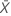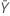Methods and formulas for Test mean / reference mean for 2-Sample Equivalence Test

The following methods and formulas are used for testing the ratio between the test mean and the reference mean.

Ratio

Notation

TermDescription
ρRatioTest meanReference mean

Means and standard deviations

The mean of the test sample,, is given by:

The mean of the reference sample,, is given by:The standard deviation of the test sample, S1, is given by:The standard deviation of the reference sample, S2, is given by:Notation

TermDescription
X iObservations from the test sample, with i = 1, ..., n1
Y iObservations from the reference sample, with i = 1, ..., n2
n1Number of observations in the test sample
n2Number of observations in the reference sample

Equivalence limits

Let k1 be the value that you specify for the lower limit and k2 be the value that you specify for the upper limit. By default, the lower equivalence limit, δ1, is given by:and the upper equivalence limit, δ2, is given by:Degrees of freedom (DF)

Do not assume equal variances (default)

By default, the degrees of freedom for the test, v, are given by the following formula:

Minitab displays v rounded down to the nearest integer.

Assume equal variances

If you select the option to assume equal variances, then Minitab calculates the degrees of freedom as follows:Notation

TermDescription
S1Standard deviation of the test sample
n1Number of observations in the test sample
S2Standard deviation of the reference sample
n2Number of observations in the reference sample

Pooled standard deviation

Notation

TermDescription
SpPooled standard deviation
S1Standard deviation of the test sample
n1Number of observations in the test sample
S2Standard deviation of the reference sample
n2Number of observations in the reference sample

Confidence interval

Minitab cannot calculate the confidence interval (CI) if any of the following three conditions are not met:

Do not assume equal variances (default)

• 100(1 - α)% CI

By default, Minitab calculates the 100(1 - α)% CI for ρ as follows:

CI = [min(C, ρL), max(C, ρU)]

where:
• 100(1 - 2α)% CI

If you select the option to use the 100(1 - 2α)% CI, then the CI is given by the following:

CI = [ρL, ρU]

Assume equal variances

If you select the option to assume equal variances, then the CI is calculated as follows.

Minitab cannot calculate the CI if any of the following three conditions are not met:

• 100(1 -α)% CI

Minitab calculates the 100(1 - α)% CI as follows:

CI = [min(C, ρL, max(C, ρU)]

Where:
• 100(1 - 2 α)% CI

If you select the option to use the 100(1 - 2 α)% CI, then the CI is given by the following:

CI = (ρL, ρU)

One-sided intervals

For a hypothesis of Test mean / reference mean > lower limit, the 100(1 - α)% lower bound is equal to ρL.

For a hypothesis of Test mean / reference mean < upper limit, the 100(1 - α)% upper bound is equal to ρU.

Notation

TermDescriptionMean of the test sampleMean of the reference sample
S1Standard deviation of the test sample
n1Number of observations in the test sample
S2Standard deviation of the reference sample
n2Number of observations in the reference sample
δ1Lower equivalence limit
δ2Upper equivalence limit
SρPooled standard deviation
vDegrees of freedom
αSignificance level for the test
t1-α,vUpper 1 - α critical value for a t-distribution with v degrees of freedom

T-values

Do not assume equal variances (default)

Let t1 be the t-value for the hypothesis,, and let t2 be the t-value for the hypothesis,, where Λ is the ratio of the mean of the test population to the mean of the reference population. By default, the t-values are calculated as follows:

Assume equal variances

If you select the option to assume equal variances, then the t-values are calculated as follows:

Notation

TermDescriptionMean of the test sampleMean of the reference sample
S1Standard deviation of the test sample
n1Number of observations in the test sample
S2Standard deviation of the reference sample
n2Number of observations in the reference sample
SρPooled standard deviation
δ1Lower equivalence limit
δ2Upper equivalence limit

P-values

The probability, PH0, for each null hypothesis is given by the following:

If, then:

H0 P-ValueNotation

TermDescription
ΛUnknown ratio of the mean of the test population to the mean of the reference population
δ1Lower equivalence limit
δ2Upper equivalence limit
vDegrees of freedom
Tt-distribution with v degrees of freedom
t1 t-value for the hypothesist2 t-value for the hypothesisNote

For information on how the t-values are calculated, see the section on t-values.

By using this site you agree to the use of cookies for analytics and personalized content.  Read our policy